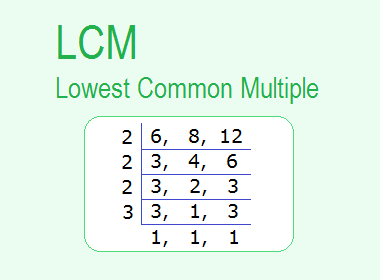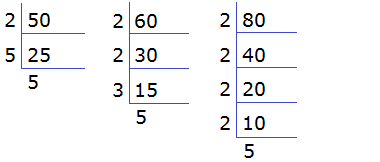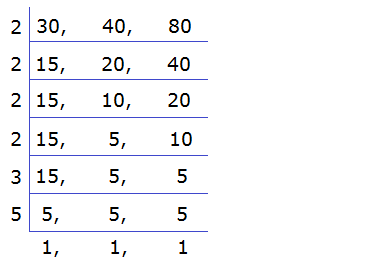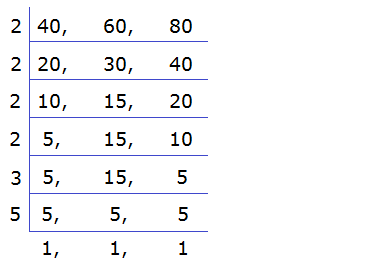# LCM (Lowest Common Multiple)## LCM (Lowest Common Multiple)

LCM (Lowest Common Multiple) of given two or more than two numbers is the lowest number which is exactly divisible by the given numbers.

********************

10 Math Problems officially announces the release of Quick Math Solver, an Android App on the Google Play Store for students around the world.

********************

Among the common multiples of the given numbers, the lowest one is the LCM.

For example, 60 is the lowest number which is exactly divisible by 10, 12 and 15. So 60 is the LCM of 10, 12 and 15.

Here,

The multiples of 10, M10 = {10, 20, 30, 40, 50, 60, 70, 80, 90, 100, 110, 120, …}

The multiples of 12, M12 = {12, 24, 36, 48, 60, 72, 84, 96, 108, 120, 132, 144, …}

The multiples of 15, M15 = {15, 30, 45, 60, 75, 90, 105, 120, 135, 150, 165, …}

Common multiples = {60, 120, …}

The lowest common multiple = 60

LCM of 10, 12 and 15 is = 60

LCM can be calculated by using the following methods:

a.     Set of Multiple Method

b.    Prime Factorization Method

c.      Common Division Method

Let's find the LCM of 12, 16 and 24 by three different methods.

### Set of Multiples Method

The multiples of 12, M12 = {12, 24, 36, 48, 60, 72, 84, 96, 108, 120, 132, 144, …}

The multiples of 16, M16 = {16, 32, 48, 64, 80, 96, 112, 128, 144, 160, 176, …}

The multiples of 24, M24 = {24, 48, 72, 96, 120, 144, 168, 192, 216, 240, …}

Common multiples = {48, 96, 144, …}

The lowest common multiple = 48

LCM = 48

### Prime Factorization Method

To find LCM by prime factorization method, we should apply the following steps:

Step 1: Find prime factors of the given numbers and list them.

Step 2: Write the common factors and the remaining factors.

Step 3: Find the product of these factors.

Here,12 = 2 × 2 × 3

16 = 2 × 2 × 2 × 2

24 = 2 × 2 × 2 × 3

The product of the common and the remaining factors is the LCM.

LCM = 2 × 2 × 2 × 3 × 2 = 48

### Common Division Method

To calculate LCM by common division method, we should apply the following steps:

Step 1: Arrange the given numbers in a row and separate by commas.

Step 2: Divide all numbers by the smallest prime numbers.

Step 3: Repeat the process till the numbers left in the last row is 1.

Step 4: Multiply all the prime divisors to get LCM.

Let’s find the LCM of 12, 16 and 24 by common division method.LCM = 2 × 2 × 2 × 2 × 3 = 48

### Worked Out Examples

Example 1: Find the LCM of 30, 40 and 60 by the set of multiples method.

Solution:

Here,

Multiples of 30, M30 = {30, 60, 90, 120, 150, 180, 210, 240, …}

Multiples of 40, M40 = {40, 80, 120, 160, 200, 240, 280, …}

Multiples of 60, M60 = {60, 120, 180, 240, 300, 360, …}

Common multiples = {120, 240, …}

Lowest common multiple is the LCM,

LCM = 120  Ans.

Example 2: Find the LCM of 50, 60 and 80 by the prime factorization method.

Solution:

Here,

The prime factors of 50, 60 and 80 are calculated as follows:50 = 2 × 5 × 5

60 = 2 × 2 × 3 × 5

80 = 2 × 2 × 2 × 2 × 5

LCM = Common factors × remaining factors

= 2 × 2 × 5 × 5 × 3 × 2

= 1200  Ans.

Example 3: Find the LCM of 30, 40 and 80 by using the common division method.

Solution:

Here,LCM = 2 × 2 × 2× 2 × 3 × 5 = 240  Ans.

Example 4: Find the least number which is exactly divisible by 12, 40 and 60 without leaving a remainder.

Solution:

Here,

The required least number is the LCM of 12, 40, and 60.LCM = 2 × 2 × 2× 3 × 5 = 120  Ans.

Example 5: Find the least number when divided by 40, 60 and 80 leaves a remainder 6 in each case.

Solution:

Here,LCM = 2 × 2 × 2× 2 × 3 × 5 = 240

The required number = LCM + remainder

= 240 + 6

= 246  Ans.

If you have any question or problems regarding the LCM, you can ask here, in the comment section below.